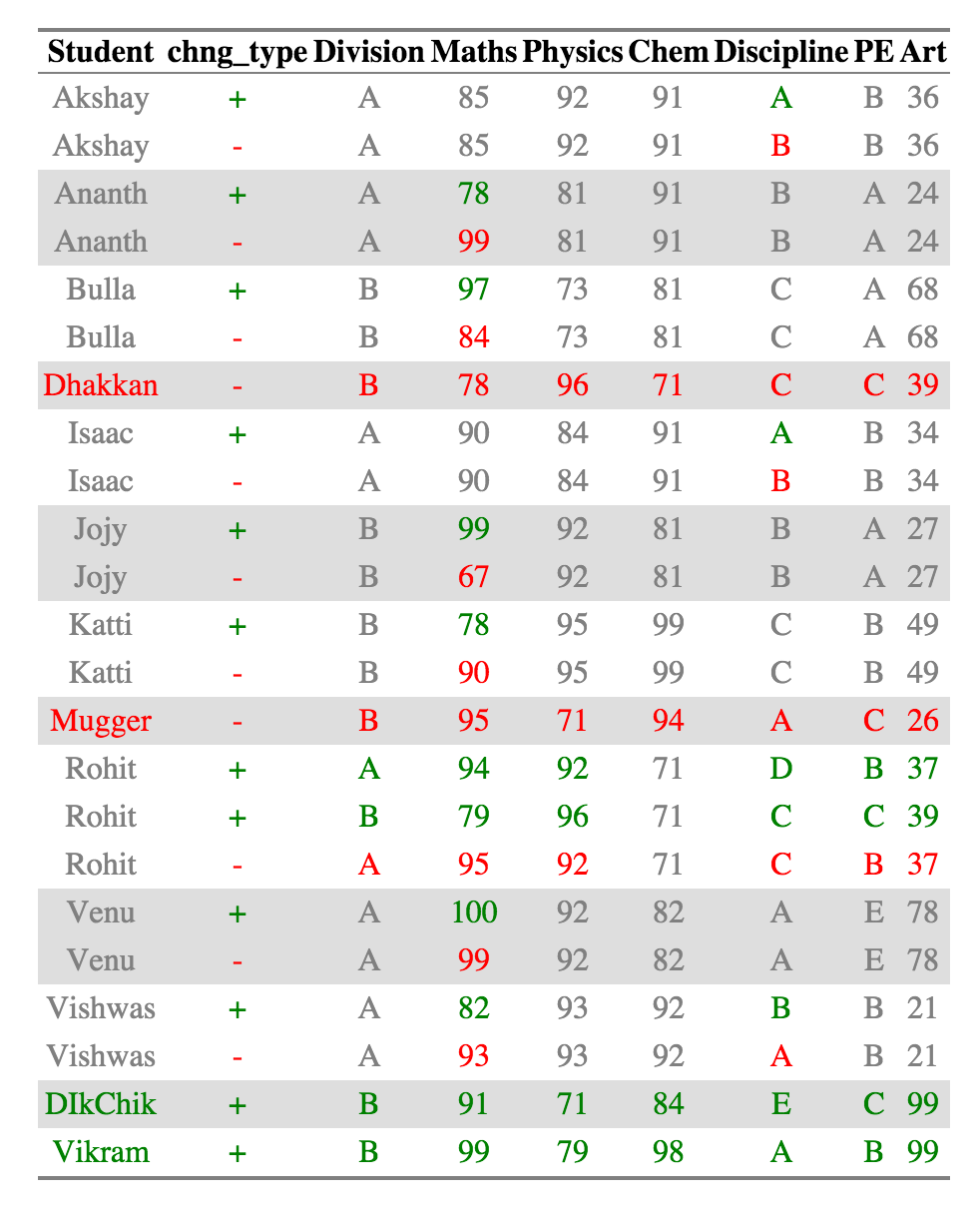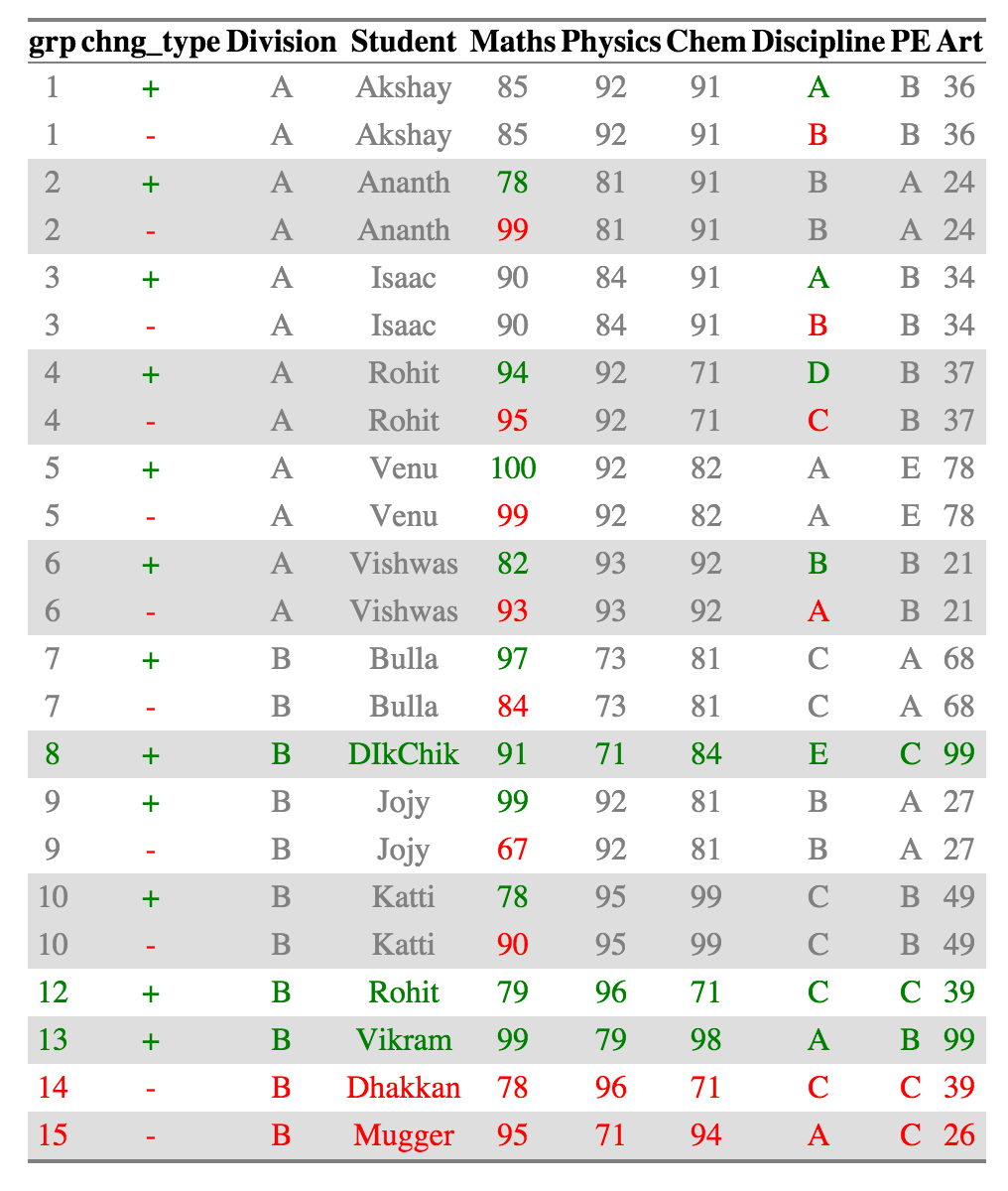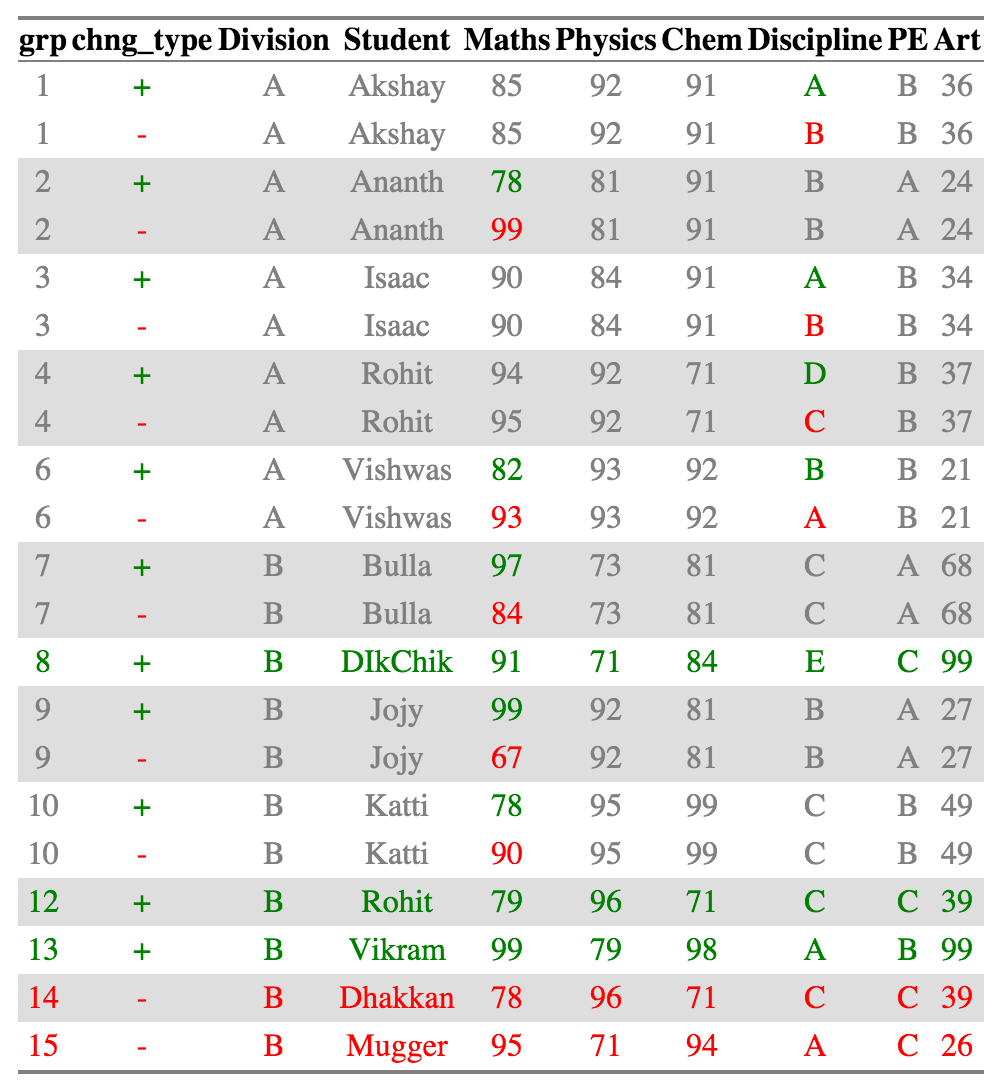CRAN Version is upto date with the latest bug fixes as of 2019-06-02

# compareDF# Introduction

Every so often while doing data analysis, I have come across a situation where I have two datasets, which have the same structure but with small differences in the actual data between the two. For example:

• Variation of a dataset across different time periods for the same grouping
• Variation of values for different algorithms, etc.

In the above cases, I want to easily identify what has changed across the two data.frames, how much has changed, and also hopefully to get a quick summary of the extent of change. There are packages like the `compare` package on R, which have focused more on the structure of the data frame and lesser on the data itself. I was not able to easily identify and isolate what has changed in the data itself. So I decided to write one for myself. That is what `compareDF` package is all about.

# Usage

The package has a single function, `compare_df`. It takes in two data frames, and one or more grouping variables and does a comparison between the the two. In addition you can specify columns to ignore, decide how many rows of changes to be displayed in the case of the HTML output, and decide what tolerance you want to provide to detect change.

# Basic Example

Let’s take the example of a teacher who wants to compare the marks and grades of students across two years, 2010 and 2011. The data is stored in tabular format.

``````data("results_2010", "results_2011")
print(results_2010)
print(results_2011)``````

The data shows the performance of students in two divisions, A and B for two years. Some subjects like Maths, Physics, Chemistry and Art are given scores while others like Discipline and PE are given grades.

It is possible that there are students of the same name in two divisions, for example, there is a Rohit in both the divisions in 2011.

It is also possible that some students have dropped out, or added new across the two years. Eg: - Mugger and Dhakkan dropped out while Vikram and Dikchik where added in the Division B

The package allows a user to quickly identify these changes.

## Basic Comparison

Now let’s compare the performance of the students across the years. The grouping variables are the Student column. We will ignore the Division column and assume that the student names are unique across divisions. In this sub-example, if a student appears in two divisions, he/she has studied in both of them.

``````library(compareDF)
ctable_student = compare_df(results_2011, results_2010, c("Student"))
ctable_student\$comparison_df
``````

By default, no columns are excluded from the comparison, so any of the tuple of grouping variables which are different across the two data frames are shown in the comparison table. The `comparison_df` table shows all the rows for which at least one record has changed. Conversely, if nothing has changed across the two tables, the rows are not displayed. If a new record has been introduced or a record has been removed, those are displayed as well.

For example, Akshay, Division A has had the exact same scores but has two different grades for Discipline across the two years so that row is included.

However, Macho, Division B has had the exact same scores in both the years for all subjects, so his data is not shown in the comparison table.

## HTML Output

While the comparison table can be quickly summarized in various forms for futher analysis, it is very difficult to process visually. The `html_output` provides a way to represent this is a way that is easier for the numan eye to read. NOTE: You need to install the `htmlTable` package for the HTML comparison to work. For the purpose of the readme I am attaching the html as a png because github markdown doesn’t retain styles.

`{r, results = 'hide'} print(ctable_student\$html_output)`Now it is very easy to see recognize what has changed. A single cell is colored if it has changed across the two datasets. The value of the cell in the older dataset is colored red and the value of the cell in the newer dataset is colored green. Cells that haven’t changed across the two datasets are colored grey.

If a new row was introduced, the Row group names (and all the other columns for that row as well ) are colored in Green. Similarly, a row group name (and the other columns in that row) are colored red if a row was removed.

For Example, Akshay, Ananth and Bulla has had changes in scores, which are in Discipline, Maths, and Maths respectively. Dhakkan and Mugger have dropped out of the dataset from 2010 and the all the columns for the rows are shown in red, which DikChik and Vikram have joined new in the data set and all the columns for the rows are in green.

The same data is represented in tabular form (for further analysis, if necessary) in the `comparison_table_diff` object

``ctable_student\$comparison_table_diff``

## Change Count and Summary

You can get an details of what has changed for each group using the `change_count` object in the output. A summary of the same is provided in the `change_summary` object.

``ctable_student\$change_count``
``ctable_student\$change_summary``

## Grouping Multiple Columns

We can also group_multiple columns into the grouping variable

`{r, results = 'hide'} ctable_student_div = compare_df(results_2011, results_2010, c("Division", "Student")) ctable_student_div\$comparison_df`Now Rohits in each individual division are considered as belonging to separate groups and compared accordingly. All the other summaries also change appropriately.

## Excluding certain Columns

You can ignore certain columns using the exclude parameter. The fields that have to be excluded can be given as a character vector. (This is a convenience function to deal with the case where some columns are not included)

## Preserving all rows

The default behavior of the `compare_df` function is to show only the records that have changed. Sometimes the use might want to preserve all the records even after the comparison and not just see the values that have been changed (especially in the HTML). In this case, you can set the `keep_unchanged` parameter to `TRUE`.

## Limiting HTML size

For dataframes which have a large amount of differences in them, generating HTML might take a long time and crash your system. So the maximum diff size for the HTML (and for the HTML visualization only) is capped at 100 by default. If you want to see more difference, you can change the `limit_html` parameter appropriately. NOTE: This is only of the HTML output which is used for visual checking. The main comparison data frame and the summaries ALWAYS include data from all the rows.

## Changing HTML color

You can use the `color_scheme` parameter to change the color of the HTML generated. The parameter must me a named vector or a list with the appropriate names - The default values are `c("addition" = "green", "removal" = "red", "unchanged_cell" = "gray", "unchanged_row" = "deepskyblue")` but can be changed as needed by the user.

## Tolerance

It is possible that you’d like numbers very close to each other to be ignored. For example, if the marks of a student vary by less that 5% across the years, it could be due to random error and not any real performance indictaor. In such a case, you would want to give a tolerance parameter. For this case, giving a tolerance of `0.05` would ignore all the changes that are less than 5% apart from the lower value.

`{r, results = 'hide'} ctable_student_div = compare_df(results_2011, results_2010, c("Division", "Student"), tolerance = 0.05) ctable_student_div\$html_output`Venu from division A who had a score change from 100 to 99 is no longer present in the diff calculation or in the output

Naturally, tolerance has no meaning for non-numeric values.

## Using compare DF in GAP analysis

The compareDF package can be used to conduct effective Gap analyses. If the package is used in this way, a configuration recommendation should be considered when using the compare_df function. Make sure the group column (group_col argument) being used as the gap classifier is unique and is not duplicated within either of the datasets being compared. Duplicate group names can skew gap analysis results. For example, using the results_2010 and results_2011 samples where Student represents the group_col argument, ‘Rohit’ group results are skewed. This is because student ‘Rohit’ is provided in the results_2011 sample twice. In this example Rohit represents two different students with the same last name. In short, if there are more than (2) records per grouping in a typical Gap analysis result, it suggests that there is a duplicate grouping contained within a single dataset that should be renamed and re-identified. If this occurs, changes should be made to the comparison datasets, and the Gap analysis should be re-conducted. The best way to prevent skewed results from occurring is to use all the columns that uniquely define a row in the group column argument - in this case, both name and student columns (alternatively a unique key identifier can also be constructed).

## Acknowledgements

Thanks to Nitin for proofreading the doc and making sure everything made sense.

## Contributors

• Brice Richard
• Joshua David Barillas - https://github.com/jdbarillas